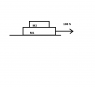# A Block On Top of A Slab

• Bashyboy

## Homework Statement

In the figure, a slab of mass m1=40kg rests on a frictionless floor, and a block of mass m2=10kg
rests on top of the slab. Between block and slab, the coefficient of static friction is μs=0.60, and
the coefficient of kinetic friction is μk=0.40. A horizontal force F of magnitude 100 N begins to
pull directly on the block, as shown.

## The Attempt at a Solution

One question I have is, what sort of friction is acting, kinetic or static? I figured that it would be static. When the force F begins pulling on m1, intertia will act to keep m2 from sliding with the slab, giving it the impression that it is moving to the left, relative to the slab's point of view. Hence, the static friction force will balance this "inertial force" that is trying to act, thereby causing the block to move in unison with the slab, or mathematically, a1 = a2.

How do I prove this mathematically, though. Do I need to compute the static friction force and compare it to some other force?

#### Attachments

•diagram.png
523 bytes · Views: 456
What is the problem? What do you have to find?

Assuming you have to find acceleration:
Have you drawn the FBDs for the blocks?
What are the forces acting on the blocks?
Also what do you mean by 'inertial force'? I think you are not clear on its definition, if so google it up!

Sorry, here are the questions:

(a) Choose the directions of your coordinate system.
(b) Identify all forces acting on the block and the slab.
(c) Write down Newton’s 2nd law for the block and the slab.
In unit‐vector notation, what are the resulting accelerations a1 and a1.

One question I have is, what sort of friction is acting, kinetic or static? I figured that it would be static. When the force F begins pulling on m1, intertia will act to keep m2 from sliding with the slab, giving it the impression that it is moving to the left, relative to the slab's point of view. Hence, the static friction force will balance this "inertial force" that is trying to act, thereby causing the block to move in unison with the slab, or mathematically, a1 = a2.

How do I prove this mathematically, though. Do I need to compute the static friction force and compare it to some other force?

The usual approach would be to make an assumption (static friction rules or doesn't rule) and see if it results in a contradiction. Probably easier to check the "static friction rules" case. So yes, compute the maximal static friction force and see if the top block's inertial force exceeds it.